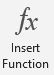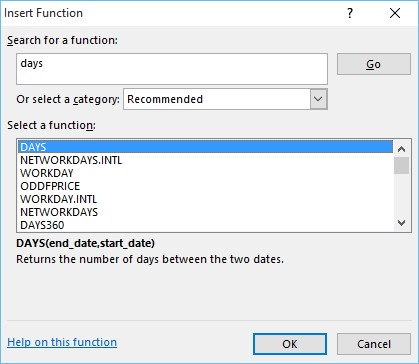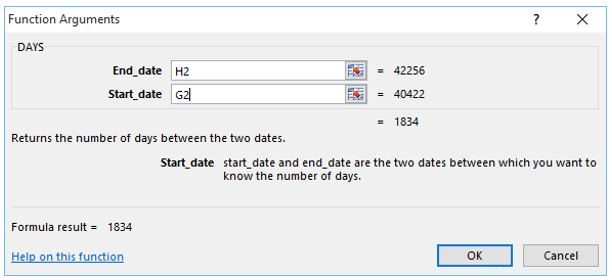Microsoft Excel 2013 has a new useful function called Days. It is pretty easy to use and only requires two arguments: an end date and a start date. The results will be displayed as the total number of days between the two dates. Follow the steps below to create the function.

1. Click in the cell you would like to return the answer in.

2. Click on the FX Insert Function button located on the Formulas tab (can also be found next to the formula bar).3. This opens up the Insert Function Dialog box. Type the word Days in the search box and click Go.

4. Select the Days function under Select a Function then click OK.5. In the Function Arguments Dialog box either enter manually the End Date and Start Date or click on the appropriate cells.6. Click OK when done.

7. The formula result will be displayed as the total number of days between the two dates.

Here is what the formula looks like: =DAYS(H2,G2).
To find the number of years, simply divide by 365: =DAYS(H2,G2)/365.

If you liked this tip, be sure to check out our FREE video on 10 AHA! Moments in Microsoft Excel.Topics: Excel